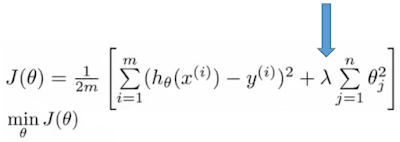# High Bias and Variance problem in Machine Learning [Cause & Solution]These days everybody talks about Machine Learning and Artificial Intelligence. More and more people started studying Machine Learning.

For beginners, Studying Machine Learning starts with understanding Linear and Logistic Regression.

While implementing Linear or Logistic Regression, we mainly face two types of problems:

## What is a high bias problem in machine learning?

When our model (hypothesis function) fits poorly with the training data trend, then we can say our model is underfitting. This is also known as high bias problem.Figure 1: Underfitted
•  High bias problem is mainly caused due to:

•  If the hypothesis function is too simple.

•  If the hypothesis function uses very few features.

•  Solution for high bias problem :

•  Adding more features to the hypothesis function might solve the high bias problem.

•  If new features are not available, we gen create new features by combining two or more existing features or by taking a square, cube, etc of the existing feature.

•  If your model is underfitting (high bias), then getting more data for training will NOT help.

•  Adding new features will solve the problem of high bias, but if you add too many new features then your model will lead to overfitting also known as high variance.

## What is a high variance problem in machine learning?

Unlike high bias (underfitting) problem, When our model (hypothesis function) fits very well with the training data but doesn't work well with the new data, we can say our model is overfitting. This is also known as high variance problem.Figure 2: Overfitted

•  High variance problem is mainly caused due to:

•  If the hypothesis function is too complex.

•  If the hypothesis function uses too many features.

The higher-order polynomial in hypothesis function creates unnecessary curves and angles in the model which is unrelated to data.

•  Solution for high variance problem :

•  Either reduce the unnecessary features from the hypothesis function.

•  Otherwise, keep all the features in the hypothesis function but reduce the magnitude of the higher-order features (terms). This method is known as Regularization. Regularization is the most widely used method to solve the problem of high variance or overfitting.

•  Regularization works well when we have a lot of slightly useful features.

•  Lambda (λ) is the regularization parameter.Equation 1: Linear regression with regularization

•  Increasing the value of λ will solve the Overfitting (High Variance) problem.

•  Decreasing the value of λ will solve the Underfitting (High Bias) problem.

•  Selecting the correct/optimum value of λ will give you a balanced

•  If your model is overfitting (high variance), getting more data for training will help.

## Summary :

•  Too simple or very few features in hypothesis function will cause high bias (underfitting) problem. Adding new features will solve it but adding too many features might lead to high variance (overfitting) problem. You can apply regularization to your model to solve the high variance problem.

•  Getting more training data will help in case of high variance (overfitting) but it won't help in high bias (underfitting) situation.

--------------------------------------------------------------------------------

Click here to see solutions for all Machine Learning Coursera Assignments.
&
Click here to see more codes for Raspberry Pi 3 and similar Family.
&
Click here to see more codes for NodeMCU ESP8266 and similar Family.
&
Click here to see more codes for Arduino Mega (ATMega 2560) and similar Family.
Feel free to ask doubts in the comment section. I will try my best to answer it.
If you find this helpful by any mean like, comment and share the post.
This is the simplest way to encourage me to keep doing such work.

Thanks and Regards,
Akshay P Daga